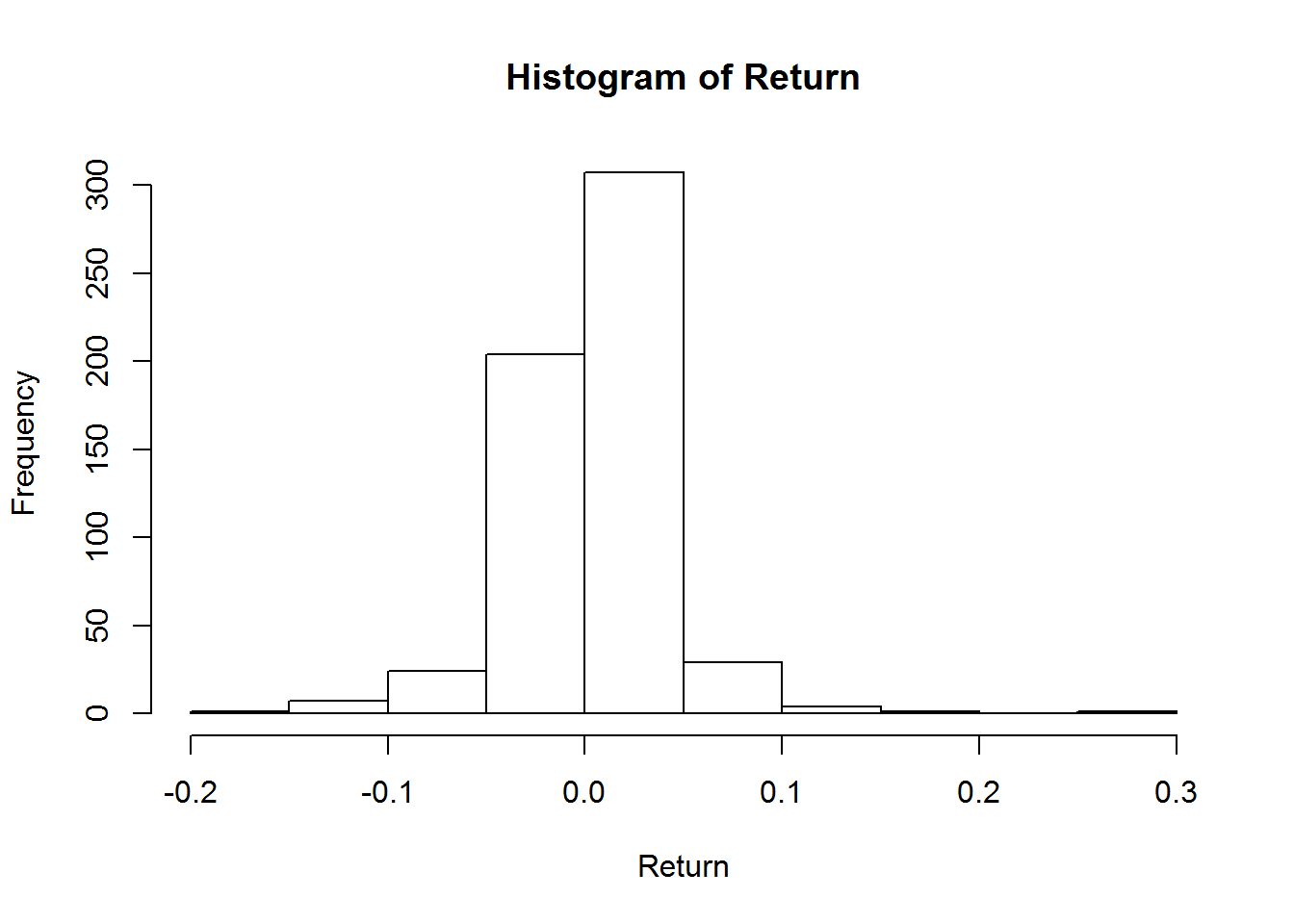# Value at Risk & Expected Shortfall of Cryptocurrencies

•
• 1
•
• 21
•
•
22
Shares

It is important to have an estimate of risk for a portfolio when trading with financial assets. Two basic and commonly used risk measures are value-at-risk and expected shortfall. We will illustrate the two concepts with an example and provide the necessary R code to actually calculate the values of both measures.

If we take the price movements of an asset and draw the distribution of returns in a diagram, we might get a picture like the one below the code which shows the distribution of returns of BTC in USD from January 1, 2016 to August 1, 2017:The left side of the histogram depicts the frequency of losses. We can see that the right side seems to have more “weight” predicting more profit on average (without considering fees,…). But what is the risk? The value-at-risk measure (see below) tells us the minimum we should expect to lose in one event within a given time period. We use the value-at-risk to calculate the expected shortfall which predicts our actual loss during that same event.

Suppose we have invested in one currency whose price movement is pi where pi is the closing price of this currency in USD at some exchange and the variable i varies over the days of a specified time period. The simple return at day i+1 is then defined as ri+1 := (pi+1– pi) / pi(Note the logarithmic return is calculated differently: log(pi+1/pi)). In the sequel, we let  r:=(r1,  …  , rn) be the vector of returns.

Suppose further that we have collected return values ri over a hundred days. The value-at-risk gives us an estimate for the value x such that 5% of the time we lose more than x. It is common to denote the value-at-risk of a return vector r by VaRα(r). So, for example, if VaR.95(r) = 0.01 then we should expect to lose more than 1% once every 5 days (note: 5 = (1 – 0.95) x 100).

Let us look at the example of BTC price in USD from January 1, 2016 to August 1, 2017 (579 days). If we again choose a 95 % confidence level, we obtain VaR.95(r) = 0.055. This result means that approximately once every 29 days (0.05 x 579), we will lose at least 5.5% in this day. You can use the following R code to determine the non-parametric VaR which is based on historical data:

The mathematical definition is: Let P be a probability density of the returns X, then the value-at-risk of level α is defined as VaRα(X):= inf { x ∈ R  | P(X<-x)  ≤ 1- α}.

Next, we can ask what loss should we expect on that day. The historical expected shortfall is just the mean of all losses which are higher than the value-at-risk. For the BTC returns example the historical expected shortfall ES.95(r) is 0.086 which means that on the day we lose more than 5.5%, we can expect to actually lose 8.6%. In R this can easily caclulated from historical data as follows:

The mathematical definition: Let X be the returns, then the expected shortfall of level  α is defined as ESα(X) := E[X | X > VaRα(X)], where E denotes the expected value. Note that the historical expected shortfall uses the mean instead of expected value.

Download the R script below to calculate the VaR and ES of your preferred cryptocurrency after downloading the necessary price charts as a csv file from CryptoCompare. To get the csv file, go to their website and choose the cryptocurrency you are interested in. Go to charts where you can download the data as a csv file. Alternatively, one could also use their API.

We close with a list of VaR and ES of some common cryptocurrencies with parameter (α = .95) for the period from January 1, 2016 to August 1, 2017 and include EUR as a benchmark. The interpretation is as above: E.g., approximately every 29 days one should expect to lose at least 6,1% of LTC, and on this specific day one should expect to lose 10%.

. BTC LTC XRP Dash ETC ETH XMR EURO
VaR 0.055 0.061 0.077 0.078 0.081 0.085 0.11 0.0083
ES 0.086 0.1 0.14 0.14 0.11 0.14 0.15 0.013

: Overview of Statistical and Extreme Value Analysis (by Osterrieder, Lorenz, and Strika)

: Coherent Measure of Risk (by Artzner, Delbaen, Eber, and Heath)

: Exploring the Limitations of Value at Rsik (by Krause)